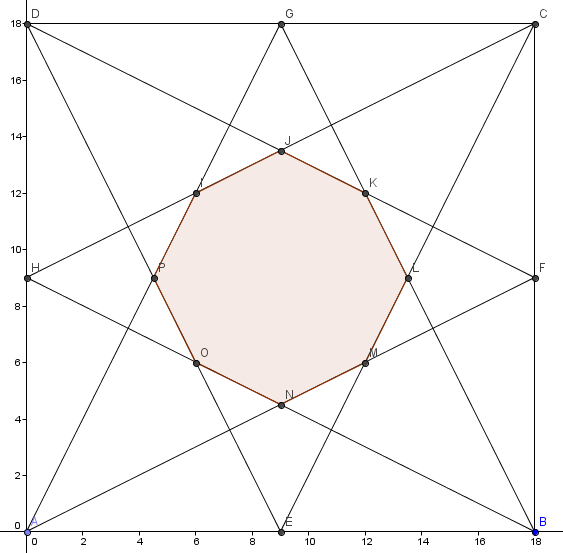# I've seen it before - 6

Geometry Level 5If $ABCD$ is a square with side $18\text{ cm}$ and $E, F, G, H$ are the midpoints of $AB, BC, CD, DA$ repectively, then join $A$ to $G$ and $F$, $B$ to $G$ and $H$, $C$ to $H$ and $E$, $D$ to $E$ and $F$. An octagon is formed from the intersection of these lines, then the area of the octagon (the shaded region in the figure) is

Note:-

• The Octagon may or may not be a regular.

• You can find more such problems here